• 最近学到目标检测识别的LBP特征，自编的程序里面有个步骤是把matlab里面矩阵列拼接成个二进制数，比如 a'=[0,1,1,0,0]; 要把a拼接转化成二进制数01100。 我的步骤是 t=''; for l=1:length(a) t=[t,num2str(a...
最近学到目标检测识别的LBP特征，自编的程序里面有个步骤是把matlab里面矩阵的一列拼接成一个二进制数，比如
a'=[0,1,1,0,0];

要把a拼接转化成二进制数01100。
我的步骤是
t='';
for l=1:length(a)
t=[t,num2str(a(1,l))];
end
num=str2num(t);

这样就得到了拼接结果01100。利用了字符串可以拼接的特点，先变成字符串，再转化成数值。


展开全文字符串 算法
• 这里主要介绍了关于矩阵的... 关于矩阵的一些操作1.1 对矩阵中的数值进行替换分析：从最开始的矩阵到后面的矩阵，第一行第二列的元素从21变成了76；第三行第二列的元素从2变成了0，只需要对这两个位置的元素重新进行...
这里主要介绍了关于矩阵的一些操作以及函数。其中关于矩阵的操作包括矩阵内元素替换、等差数列产生、清除矩阵的某行或某列、两个矩阵组合成为一个大矩阵。关于矩阵的函数包括特殊矩阵生成函数以及对矩阵运算的一些特殊的函数。 1. 关于矩阵的一些操作1.1 对矩阵中的数值进行替换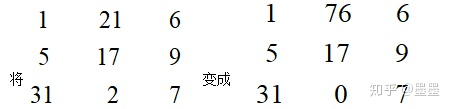分析：从最开始的矩阵到后面的矩阵，第一行第二列的元素从21变成了76；第三行第二列的元素从2变成了0，只需要对这两个位置的元素重新进行幅值就可以完成矩阵中数值替换。具体MATLAB实现如下：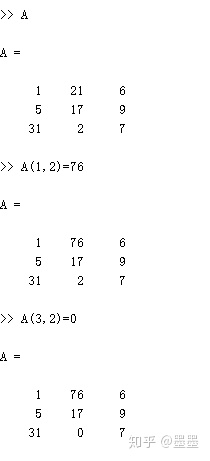1.2  等差数列的建立如果要建立一个数列为1 2 3…100差值为1，从1到100语句为：A=1:100如果是建议数列为 1 3 …99那么就是差值为2，从1到100语句为B=1:2:1001.3 将矩阵的某行或者某列元素清除形成新的矩阵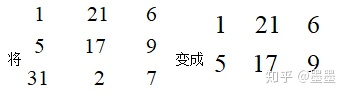分析：上面的变化是将矩阵的第三列去掉了。A矩阵的第三列表示为(3,:)，将它赋值为[ ]即可。MATLAB实现如下：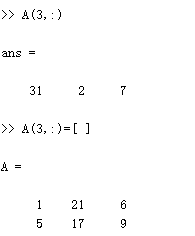1.4 两个矩阵组成大矩阵如果两个矩阵之间使用的是空格，那么会进行行扩展；如果两个矩阵之间使用的是分号，会进行列扩展。MATLAB实现如下：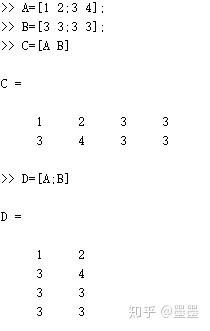2. 一些特殊的矩阵生成函数① zeros(m,n)：产生m行n列的0矩阵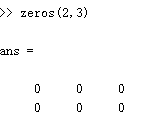② ones(m,n)：产生m行n列的1矩阵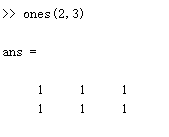③ eye(n)：产生单位矩阵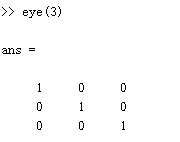④ diag()：产生的是对角线矩阵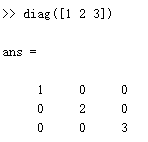⑤ rand()：产生的是随机数矩阵，数值为0到1中的数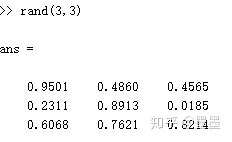3. 有关矩阵的运算函数① max(A)、max(max(A))、min(A)、min(min(A))函数：如果是用max(A),会显示出矩阵中最大元素的那一行；如果使用max(max(A))则会显示矩阵中最大的那个元素，min函数与上面的类似。具体看下面的例子：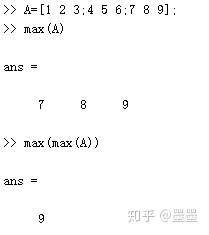② sum(A)、sum(sum(A))、mean(A)、mean(mean(A))函数：Sum(A)是将每一列的元素进行求和得到结果sum(sum(A))是将所有元素进行求和，而mean是求均值，具体用法与sum函数类似，具体举例实现如下：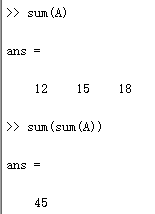③ sort(A)、sortrows(A)函数：这两个是排序函数Sort(A)表示对每一列的元素在列内进行排序，sortrows(A)则是对矩阵中行元素和的大小进行排序，具体实现如下：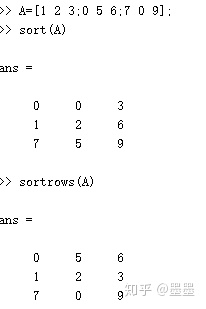④size(A)与length(A)函数：size(A)是计算A矩阵的行列的个数，实现结果如下：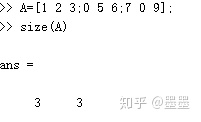length(A)表示数组的长度，实现结果如下：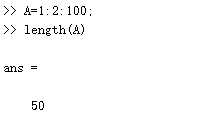⑤ find(A)函数：find函数是寻找矩阵中的元素位置，具体实现如下：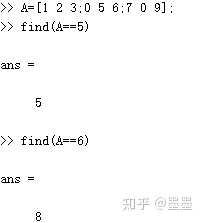结果表示：5这个元素在矩阵中第五个位置；6在矩阵中第八个位置
展开全文• 用基础的函数写了matlab脚本将矩阵变成行最简型。未参考matlab自带的rref函数，基本与其无关，如有bug可反馈 function R = My_RS(A) %A表示输入矩阵 %R表示输出的最简型矩阵 t = 1; T = zeros(size(A...
用基础的函数写了一个matlab脚本将矩阵变成行最简式。未参考matlab自带的rref函数，基本与其无关，如有bug可反馈。
大致思路:把矩阵含有0的行放在最下面，然后下面的行减去上面的行，最后变成行最简型。
function R = My_RS(A)
%A表示输入矩阵
%R表示输出的行最简型矩阵

t = 1;%进行行循环的元素
T = zeros(size(A, 1), size(A, 2));%设定一个T矩阵，用来排序的
i = 1;%进行列循环的元素

while i < size(A, 2)
c = size(A, 1); flcg = 0; sf = 0; flgg = 0;
%将A进行降序排序，并且把含有0的列放在最后
A = sortrows(A, 'descend');

for j = t:size(A, 1)
v = j;
op = 0;

for k = t:size(A, 1)

if A(k, i) == 0
op = op + 1;
end

end

if A(j, i) == 0

for l = v + op:size(A, 1)
T(v, :) = A(v, :);
A(v, :) = A(l, :);
v = v + 1;
end

for w = size(A, 1) - op + 1:size(A, 1)
A(w, :) = T(w, :);
end

break
end

end

%进行行循环
for j = t:size(A, 1)

if A(j, i) == 0
c = j - 1; break
end

end

%将t行i列下的元素变为0
if c - t >= 1

for m = c:-1:t + 1
%判断两个值是否近似，以方便进行加减计算
for cc = i:size(A, 2)

if abs(A(m, cc) - A(t, cc)) < 10e-10
A(m, cc) = A(t, cc);
end

end

A(m, :) = A(m, :) - (A(m, i) / A(t, i)) .* A(t, :);
end

end

%判断t行前i列是否都为0
if i > 1

for gg = i - 1:-1:1

if A(t, gg) ~= 0
flgg = 1;
break
end

end

end

if flgg == 0
%第一个元素变为1
if A(t, i) ~= 0
A(t, :) = A(t, :) / A(t, i);
end

%将t行i列上的元素变为0
if t >= 2

for v = 1:t - 1
%判断是否近似
for dd = i:size(A, 2)

if abs(A(v, dd) - A(t, dd)) < 10e-10
A(v, dd) = A(t, dd);
end

end

A(v, :) = A(v, :) - (A(v, i) / A(t, i)) .* A(t, :);
end

end

end

%判断下一列(下几列)t行下的元素是否全为0
if i < size(A, 2) && t < size(A, 1)

for f = i + 1:size(A, 2)

for s = t + 1:size(A, 1)

if A(s, f) ~= 0
flcg = 1;
break

end

end

if flcg == 1
break
else
sf = sf + 1;
end

end

end

%跳过下一列(下几列)
if flcg == 0
i = i + sf;
end

if t < size(A, 1)
t = t + 1;
else
break
end

i = i + 1;
end

%赋值结果
R = A;
end



展开全文c语言
• 考虑这个问题：定义个简单的向量a 如何复制10呢？即： 同理，对于个列向量，如何复制 10 列呢？ 　关键函数１： repmat( A , m , n )：将向量／矩阵在垂直方向复制m次，在水平方向复制n次。 再举...
考虑这个问题：定义一个简单的行向量a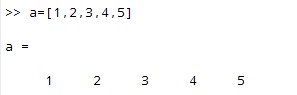如何复制10行呢？即：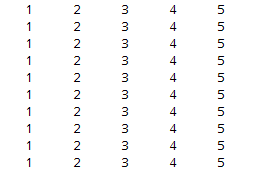同理，对于一个列向量，如何复制 10 列呢？

关键函数１： repmat( A , m , n )：将向量／矩阵在垂直方向复制m次，在水平方向复制n次。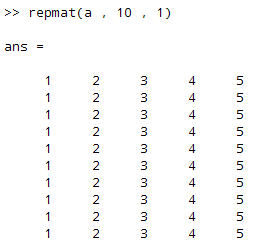再举一个例子，对于 a =[1 2;3 4]: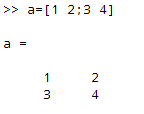垂直方向复制３次，水平方向复制２次，结果是：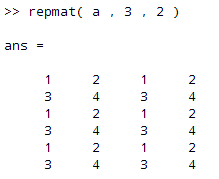接下来进阶一下，玩点复杂的。对于 a = [1 2;3 4]，如何变成下面这样：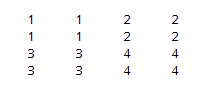关键函数: kron( a , B )　：对 a 的每一个元素 e，都数乘B矩阵，构成新的矩阵。具体用法可以doc kron。

引用 Matlab 的帮助文档，对于 A 和 B，kron(A,B) 的图示如下：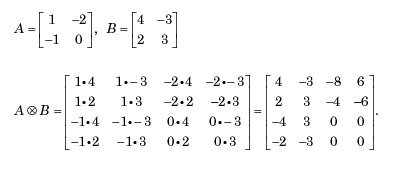回到上面的问题，对于 a=[1 2;3 4]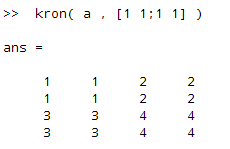展开全文• 这里主要介绍了关于矩阵的... 关于矩阵的一些操作1.1 对矩阵中的数值进行替换分析：从最开始的矩阵到后面的矩阵，第一行第二列的元素从21变成了76；第三行第二列的元素从2变成了0，只需要对这两个位置的元素重新进行...
• 应用实例 A = [1,2,3;4,5,6;7,8,9] B = A(:) C = A(:)' 结果： ...如果是以行数据的方式排成一行或一列，可以将A倒置。（也许有可以直接使用的程序，目前还没有找到，暂时这样使用。） 结果如下：
• 基础资料参考：...补充： max(a)，默认按列比较 max(a,[],1),按列比较 max(a,[],2),按比较 a(:),变成全是矩阵 max(max(a)),获得最大值 [i,j]=max(a(:)),i是最大值，j是索引 min同理 sort(A, 1...
• 1.置换矩阵维度 permute 比如矩阵为13*11*4，想变成11*13*4 permute(A,[2,1,3]) 2.交换两列 交换第列和第四列 A(:,[1,4],:)=A(:,[4,1],:)
• matlab提取语音的mfcc特征得到个[nx24]的矩阵，但是每个语音的帧数都不一样，所以我想把这些[nx24]的矩阵变成【1x24】的向量，不知道有什么办法可以处理，而且变成【1x24】的矩阵后还能保持它的特征python 机器学习 深度学习
• 第三步输入 A.’，对矩阵进行转置，可以看到23列的矩阵变成了32列的矩阵，行列变换，如下图所示： matlab中怎么求矩阵的转置 第四步我们也可以看一下共轭转置，输入A’，是相同的结果，如下图所示： matlab中...
• %排序后变成1001列向量 [m,n]=find(A<=t(20),20);%在A矩阵找到小于t(20)的20个数 index=[m,n]%前20个最小项在矩阵A中的位置[,列] 转载于:https://www.cnblogs.com/litthorse/p/9265936.html...
• 任意矩阵都能通过初等变换变成行阶梯形，这个阶梯型所含非零的行数就是矩阵的秩，矩阵的秩是矩阵个数字特征，是矩阵初等变换的个不变的量，对于矩阵研究具有重要意义。 matlab 求法： 给出矩阵a ...
• 假设a=rand(2,3,4) %产生了23列子数组4个 b=a（:,:,1）%取第一个2*3数组再如：a=rand（784，...b=reshape（b,28,28） %变成一个28*28矩阵 imshow（b）可以看到一个图像内容 b=reshape(b,784,1) %又变成了一个列向量。reshape
• 这里主要介绍了关于矩阵的一些操作以及函数。其中关于矩阵的操作包括矩阵内...分析：从最开始的矩阵到后面的矩阵，第一行第二列的元素从21变成了76；第三行第二列的元素从2变成了0，只需要对这两个位置的元素重新...
• ## matlab小技巧 续行符

万次阅读 2007-11-14 18:49:00
续行符就是...三个英文的点比如有30个数据，希望输入5个数据就另起移行，这样看上去比较工整但直接输入回车或shift+回车，会使得输入的数据变成6X5矩阵了在每一行后输入...就可以保持原来1X30的向量形式
• 现在我想设一个2×1的矩阵X(n)，第一行是i(n)，第二行是u(n)，请问大佬们要如何设？ 如果直接设置X(n)=[i(n);u(n)];就会说 无法执行赋值，因为左侧的索引与右侧的大小不兼容 如果设成X{n}=[i(n);u(n)];那X就...开发语言
• 这种办法确实能删除NaN，但是会把原来的数组变成列向量，损坏了原来的结构。 在结构不遭破坏的基础上，删除掉NaN的位置。此方法需要保证对于每列而言，NaN所在的索引是相同的。为保证这个条件在导入前需要观察数据...
• 设A为34列的矩阵，B为个行数大于3的矩阵，写出MATLAB命令。 （1）删除A的第1、3两列。 （2）删除B的倒数第3。 2解答 A = [1:4;5:9;10:14] B = rand(4) A(:,[1,3])=[] B(end-2:,)=[] 例题 例1 建立个...
• ## LU分解(matlab实现)

万次阅读 多人点赞 2016-05-02 17:32:05
主要的算法思路是从下至上地对矩阵A做初等变换，将对角线左下方的元素变成零，这些变换的效果等同于左乘系列单位下三角矩阵，而变换得到的上三角矩阵就是U. 分解算法如下：（其中 alpha 代表 L矩阵的元素，...LU分解
• 1，在Matlab基本的数据结构就是矩阵，而所说的的标量（1*1的矩阵），向量（向量，列向量），数组（与向量是同种数据结构，只是向量是数学中的叫法，而数组就变成语言中的叫法），其实就是特殊的矩阵。...
• Matlab与机电系统仿真二 ------变量的赋值 变 量 在Matlab中我们给每个数据标量向量矩阵个名字以便我们随时实用数据这个名字叫变量 例 m=5.6; %Mass of a particle g=-9.8; %Gravity acceleration G=m*g; %...
• 可能会变成一个梯形。切向畸变可以通过如下公式来校正： xcorrected = x + [ 2p1y + p2 (r2 + 2x2) ] ycorrected = y + [ 2p2x + p1 (r2 + 2y2) ] 这里（x, y）是畸变点在成像仪上的原始位置，r为该点距离成像仪中心...
• 将A变成m*n型矩阵 rot90(A);将A逆时针旋转90度 fliplr(A);左右翻转 flipud(A);上下翻转 size(A);求矩阵A的尺寸大小，返回值为A的行数和列数，故前面的返回值应为两个。另外，a=A(m,:);选出A的第m b=A(:,n);...
• 这个程序就是,首先导入一张图片,先将图片转换成灰度图,然后把一行两列第一副图显示出来,再把第一行第二列的第二张图片以灰度直方图显示出来 将图像分解为R,G,B图像后,再对分解后的二维图像求其R,G,B的直方图 ...
• 1.[A;B]=a1 a2 a3 ......b1 b2 b3......; 的作用是产生一个矩阵。2. [abc,123]=abc123, 的作用是 拼接 ，类似...3.[A B]=[a1 a2 a3 ...b1 b2 b3...]无符号的意思是将另两个1*n矩阵变成一个1*2n的向量（矩阵）。...
• 举个例子，x=[1,2,3,4]，y=[3,2,4,1]，我想让y按从小到大的顺序排列，但x...只要先将两个数组放入到矩阵中，xy=(x,y)，然后用sortrows()函数就好，xy=sortrows(xy,2)。 这个方法不知道不行 但是 有种方法 很
• 有的同学希望能将这个对角线元素单独抽取出来变成一向量或者列向量，还希望特征值从大到小排列，为了达到这个目的，大家用了各种各样的方法。其实 MATLAB 内置的函数就有diag()可以将一个对角矩阵转化为一个向量...# matlab矩阵变成一行matlab 订阅